# Fabrication, Characterization, Regeneration and Application of Nanomagnetic Fe3O4@Fish Scale as a Bio-adsorbent for Removal of Methylene Blue

Document Type : Original Research Paper

Authors

1 Chemical Engineering Department, Engineering Faculty, University of Guilan, Rasht, Iran

2 ouman Faculty of Engineering, College of Engineering, University of Tehran, Fouman, Iran

Abstract

The methylene blue (MB) adsorption from aqueous solution was investigated through Fe3O4 nanoparticles loaded on Fish Scale (FS) from fishery biomass. The presence of collagen fibers, apatite crystals and nano-magnetite particles in the structure of nano-magnetic fish scale (MFS) was observed in FTIR, EMA and XRD results. From nitrogen physisorption studies, the FS and MFS specific surface areas were estimated at 0.65 and 4.86 m2/g, respectively. The negative values of ∆G0 and ∆H0 confirmed that the adsorption was a spontaneous and exothermic process, respectively. The MB adsorption onto MFS was a physisorption controlled process. The Sips equation estimated the best fit to the data compared with other isotherm equations. The Langmuir and Sips maximum adsorption capacities (Qmax) were 68.72 and 60.87 mg/g, respectively. MB removal by MFS followed the model of pseudo-second order rate kinetics. The reusability potential of the MFS was studied, and results showed an efficiency of 59.63%.

Keywords

#### Full Text

INTRODUCTION

In all previous studies, a roughly similar method has been used for the application of FS (without modification). A nano-magnetic adsorbent was produced by modifying the surface of common carp FS with Fe3Onanoparticles in order to assess its capacity as an adsorbent to remove MB dye in a batch system. This is the first research on adsorption of a cationic dye by an FS based adsorbent modified by Fe3O4 nanoparticles. First, the structure and morphology of the magnetic FS were specified using Fourier transform infrared spectroscopy (FTIR), nitrogen physisorption and field emission scanning electron microscopy (FESEM). Then, all controlling factors and conditions of optimization was explored and evaluated which are reaction contact time, initial dye concentration, pH, and the regeneration efficiency of the magnetic adsorbent.

EXPERIMENTAL SECTION

Materials

The FSs were obtained from the fish bazaar in Rasht, Guilan Province, Iran. Common carp was chosen among the many kinds of fishes used in the north of Iran because of its abundance and favorable scale size. MB was purchased from Merck (C16H18N3SC, M=319.8 g/mol, density=1.757 g/mL), and chosen as a representative reactive dye. An MB stock solution was provided via dissolving 1.0g of MB in distilled water (1 L), and the different MB solutions (50–500 mg/L) were obtained by diluting the stock solution. The solution pH was set to the desired value via adding 0.1 M and/or 0.01 M of HCl or NaOH. FeSO4.7H2O (M=278.02 g/mol, density=1.895 g/mL) and FeCl3.6H2O (M=270.33 g/mol, density=1.82 g/mL) were purchased from Ghatran Shimi Co., Iran.

Initially, the FSs were entirely rinsed with tap water, and then they were washed with sodium hypochlorite solution (5% wt) to eliminate any adhering dirt; simultaneously they were deodorized and degreased. Finally, the raw materials were rinsed with distilled water and were dried at 80 °C for ۲۴ h. Finally, the FSs were crushed, sieved into 100–500 μm size range, and preserved in desiccators (Fig. 1a).

Synthesis of Nano-Magnetic FS

The technique of chemical precipitation was applied to provide particles which have a narrow size distribution and homogeneous composition. 3.2 g of FeSO4.7H2O and 6.2 g of FeCl3.6H2O (Fe (III): Fe (II) molar ratio was 2:1) were dissolved in 160 mL of distilled water with severe stirring and an inert atmosphere to obtain 4 g of magnetic particles, 20 mL of ammonium hydroxide solution (25 %) was added while the solution was getting heated to 80 °C. Next, as the solution color varied from light brown to black, the solution was left for 10 min to make sure about the ultimate growth of the crystals of the nanoparticle. Afterward, 20 g of pulverized FS was added to the solution, and the reaction was being done for 70 min at 80±1 °C with continuous stirring. Finally, the suspension was cooled to room temperature, and then it was washed several times using distilled water to eliminate chemicals which were un-reacted. Magnetic nanoparticles of Fe3O4–fish scale (MFS) adsorbents were tested with a magnetic rod, and the synthesized adsorbents (MFS) were attracted to the magnetic rod, which could be due to the iron’s magnetic behavior (Fig. 1b,c). The reactions occurring in MFS generation are shown as follows: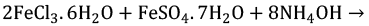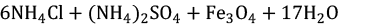(1)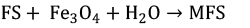(2)

Characterization Instruments

FTIR (Bruker, USA, Alpha) of the dried MFS, before and after MB adsorption, was done in the wavenumber range of 400-4000 cm-1 for determination of the binding groups’ type on the adsorbent by employing KBR pallet pressed disk technique. The area of the specific surface on the basis of nitrogen physisorption was estimated by the Brunauer–Emmett–Teller (BET) theory. The morphology and surface structure of FS and MFS were specified by utilizing FESEM at a 20 kV acceleration voltage before and after MB adsorption (FESEM, TESCAN-VEGA, Czech Republic). The overall chemical composition was determined by an EDX spectrometer (Oxford instruments-INCA, England) coupled to the FESEM. Elemental distribution spectroscopy (EDS) and elemental distribution mapping (EDM) analysis were also performed. The adsorbents’ XRD patterns were achieved by a diffractometer (SIEMENS, D5000, GERMANY, λ=1.54059 ) with Cu anode and linear focus (40mA and 40kV) in steps of 0.03° from 2θ=10° to 70° for studying the nature of adsorbents.

Point of Zero Charge (pHpzc)

The point of zero charges (pHpzc) was specified by using 0.01 M NaCl solution. First, the NaCl solutions’ pH was set at 3-11 via adding adequate ate value of 0.01 M and/or 0.1 M NaOH or HCl solution. Second, 0.1 g of adsorbent was added to the solutions; then, the solutions’ final pH was obtained after 48 h. The pHpzc was obtained from the intersection point of the curve ofpHfinal vs. pHinitial.

In order to determine the effect of contact time and the equilibrium time, batch kinetic experiments were done by adding a fixed amount of FS and MFS (0.3 g) into 50 mL of the MB solution at constant initial concentration (200 mg/L) at 303K and pH of 8 and shaking for predetermined intervals of time (up to 160 min). The each MB solution concentration at different time intervals was determined by using a UV–vis spectrophotometer (Rayleigh UV-2601, China). Also, optimum adsorbent dosage was determined with adding different amounts of MFS (0.05–0.5 g) into the 50 mL MB solution (200 mg/L) with a constant pH of 8 at 303K.

Equilibrium and Kinetic Studies

The effect of MB solution initial pH on adsorption of MB was assessed by dissolving 0.3 g of MFS to the MB solutions (50mL-100 mg/L) with various initial pHs of 3.3, 5.2, 7, 8.9, and 10.9 at 303 K. Equilibrium evaluations were done through adding a constant value of MFS (0.3 g) with 50 mL of the MB solutions by various initial concentrations (50, 100, 200, 300, 400, and 500 mg/L) at 303, 313 and 323 K and pH of 8. The concentration of MB solutions was indicated using spectrophotometry at the peak absorbance wavelength (665 nm) by a double beam UV–vis spectrophotometer. Adsorption kinetics experiments were done via dissolving 100 mL of the MB solution with various initial concentrations (50, 100 and 200 mg/L) with 0.6 g of MFS at room temperature. The MFS equilibrium adsorption capacity was calculated as follows .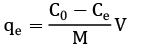(3)

Where qe is the dye amount taken up by the adsorbent (mg/g) at equilibrium; C0 is the initial concentration of dye (mg/L); Ce is the equilibrium concentration of dye (mg/L). V is the dye solution volume (L), and M is the adsorbent’s mass (g).

The data of equilibrium isotherm were consistent with some popular nonlinear two /three parameters- isotherm models included Langmuir, Freundlich, Sips, and Redlich-Peterson by using the curve fitting toolbox of MATLAB software (R2014a). The Langmuir isotherm can be expressed as follows :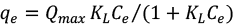(4)

Where qe (mg/g) is the adsorbed amount of dye, Qmax (mg/g) is the Langmuir maximum adsorption capacity, KL (L/mg) is the Langmuir adsorption constant, and Ce (mg/L) is the equilibrium concentration of the dye in solution. The Freundlich isotherm can be expressed as follows :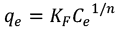(5)

Where KF (mgg-1(mgL-1)-1/n) and n are fitting constants which can be related to capacity and strength of adsorption, respectively. The Sips and Redlich-Peterson three-parameter isotherms can be written in the following forms, respectively: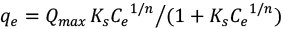(6)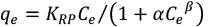(7)

Where Ks ((mg-1)-1/n) and n are fitting constants of Sips isotherm, KRP (Lkg-1) and α (kgmg-1) are fitting constants of Redlich-Peterson, and β is basically in the range of zero to one. The appropriateness of these models was assessed using a non-linear correlation coefficient (R2) .

The dynamics of MB adsorption was studied using the linear form of pseudo-first-order and the pseudo-second-order models, which were represented by the following equations, respectively [5,19]: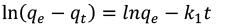(8)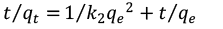(9)

qe and qt are the adsorption capacities of MFS (mg/g) at equilibrium and any instant of time, respectively. k1 and k2 (1/min) are the rate constant of pseudo-first order and pseudo-second order (g/mg.min), respectively. The plots’ slope and intercept between ln(qe-qt) vs. t and t⁄qt vs. t give the values of k1 and k2, respectively.

Regeneration Study

5g of used-MFS (UMFS) was initially washed with tap water and then treated with HCl (0.1 N, 500 mL) for 24 h to study the reusability capacity of the UMFS. Finally, it was extensively washed using distilled water several times so that neutral pH was obtained. Since desorption of MB is favorable at low pH values, HCl can be appropriate for regeneration purpose. The regenerated MFS (RMFS) was dried and stored for further experiments. The equilibrium studies were conducted with the same conditions of main experiments (MFS adsorbent experiments) at 303 K.

RESULTS AND DISCUSSION

Characterization

FTIR Analysis

The MFS’s and UMFS’s FTIR spectra are depicted in Fig. 2a and b. In summary, the FTIR absorption bands of PO43- (1038 cm-1) (Nadeem et al., 2008)and CO32 (875, 1448, and 1544 cm-1) [16,20] can be associated with hydroxyapatite and carbonated apatite in FS, respectively (Fig. 2a). Generally, FSs are composed of hydroxyapatite and collagen . The presence of collagen in the structure of adsorbent can be approved by five main bands of absorption at 1544 cm-1 (amide II), 1239 cm-1 (amide III), 1639 cm-1 (amide I), 3419 cm-1 (amide A), and 2930 cm-1 (amide B) . The peak at 605 cm-1can be due to the presence of Fe–O group, which is related to the Fe3O4 nanoparticles on the adsorbent . The peak at 3419cm-1 was assigned to water (H2O), and a small band at 2364 cm-1 was attributed to potassium bromide (KBr), which can be related to KBr pressed disk method of FTIR , which was mentioned in characterization instruments section. The FTIR spectrum of UMFS in Fig. 2b shows that after MB adsorption, some structures such as collagen (1239 cm-1), hydroxyapatite (1038 cm-1) and carbonated apatite (1544 cm-1) have been weakened or removed from the adsorbent, which can be due to an adsorbate-adsorbent interaction in the MB solution.

Nitrogen Physisorption (BET Isotherm)

Based on the BET isotherm, the FS’s and MFS’s specific surface areas were obtained at 0.65 and 4.86 m2/g, respectively. The surface area of the magnetic adsorbent (MFS) was more than seven times greater than before the loading of nanomagnetic particles (FS) on it. However, Ribeiro et al , and Ho et al.  reported the specific surface area (BET method) of Oreochromis niloticus and Malaysia local fishes equal to 2.6 and 0.15 m2/g, respectively. It is clear that loading of Fe3O4 nanoparticles on FS has a great impact on the improvement of its specific surface area.

ESEM Analysis

The FESEM micrographs of FS, MFS, and UMFS are shown in Fig. 3 with double magnifications. The surface of FS was found to be homogeneous with tiny cracks (Fig. 3a and a´), by which it’s non-porous morphology was confirmed, and also has identical and arranged growth rings which were distributed on the adsorbent surface . Therefore, FS is solid along with a rare value of mesopores which tends to be a non-porous adsorbent. However, after the loading of Fe3Onanoparticles on FS, the surface of adsorbent showed heterogeneous and porous structure (Fig. 3b and b´). Different morphological patterns become clear by comparing Fig. 3a and b. Some of the Fe3O4 nanoparticles are shown by crystal diameter with yellow lines in the Fig. 4. Since the crystal diameters are less than 100nm, it can be approved that Fe3O4 nanoparticles have been successfully synthesized on FS. A complete surface coverage of adsorbent by MB is shown in Fig. 3c and c´. The surface of the adsorbent was homogenized, smoothed and less porous after adsorption of MB. The FESEM images after the regeneration process are shown in Fig. 3d and d´. It can be seen that the uniform layer of dye molecules on UMFS has mostly been cleared from the adsorbent surface, and the initial adsorbent’s mesoporous structure became apparent.

EDX, EDS and (EMA)

The overall elemental chemical analysis of FS, MFS, and UMFS was identified and qualified using EDX (Table 1) and EDS (Fig. 5). The existence and the atomic percent of the elements, which are reported in Table 1 and Fig. 5, respectively, can render a superior perception of the adsorption process. Some of the most important ones are discussed below:

a) Cl element: Presence of Cl element may be related to the pretreatment of FS by sodium hypochlorite, which is mentioned in the preparation of initial adsorbent section. The slight atomic percent of Cl reported in Table 1 can confirm the latter conclusion. The atomic percent of Cl decreased in MFS and UMFS because of Fe3Oloading and adsorption of MB, respectively. The reason for the increase in the atomic percent of Cl in the regenerated adsorbent (RMFS) will be explained in the regeneration section.

b) Fe element: As it is obtained from Table 1 and Fig. 5, there is no Fe element on FS before modification by Fe3O4 nanoparticles and, as a result, the presence of Fe in MFS can approve the loading of Fe3Onanoparticles onto FS. The atomic percent of Fe decreased in UMFS because of the adsorption of MB. The reason for the increase in the atomic percent of Fe in RMFS will be explained in the regeneration section. The EMA analysis of Fe element on the surface of MFS is shown in Fig. 6a, where red points represent the Fe element.

c) N and S elements: The FS composition mainly includes C, O, P and Ca elements . It initially does not have any N or S element (Table 1). Since N and S elements are included in the structure of MB molecule (C16H18N3SC), the presence of N or S element in the composition of UMFS (Table 1 and Fig. 5c) can confirm the adsorption of MB on FS. The EMA analysis of N element on the surface of UMFS is shown in Fig. 6b, where red points represent the N element.

XRD Analysis

The patterns of XRD of FS and MFS powders are displayed in Fig. 7. The diffraction peaks for FS are at 2θ = 26°, 32°, 40°, 47°, 49.5°, 54° and 64° (Fig. 7a). As previously mentioned, FS mainly consists of hydroxyapatite; therefore, this peaks’ composition is in agreement with the powder of hydroxyapatite from crystallography open database . According to the XRD pattern of synthesized Fe3O4 (data not shown), the diffraction peaks for Fe3O4 crystals are at 2θ=30.2°, 35.6°, 43.5°, 54.3°, 57.4° and 63.1° . Consequently, loading of Fe3Onanoparticles on FSs can be approved by the presence of three peaks, which are shown with black circles on the XRD pattern of MFS (Fig. 7b), but not on the XRD pattern of FS. The broad crystalline diffraction around 2θ=20° represents the amorphous structure of the collagen .

Operating Parameters

Determination of the pH of Point of Zero Charge (pHpzc) and the Effect of the Initial pH of Solution

An influential factor of pH-sensitive adsorbents is pHpzc, which specifies the linearity of pH sensitivity domain and then determines the surfaces’ adsorption capabilities and the type of surface active centers. At pHpzc the total surface positive charge is in equilibrium with the overall negative charge of the surface. Also, the surface is charged positively below pHpzc, while at pH values above pHpzc, the surface is charged negatively . Adsorption of Cationic dye is desired at pH > pHpzc because of functional groups including groups of hydroxyl and carboxyl; and conversely, at pH < pHpzc, adsorption of anionic dye is favored while the adsorbent surface becomes charged positively . The pHfinal versus pHinitia graph was drawn (Fig. 8a). The curves’ intersection with the straight line for pHfinal vs. pHinitia gives the pHpzc, and the amount is 7.2 for MFS. Fig. 8b also presents the influence of initial pH of MB solution on the removal of MB. Fig. 8b shows that the MB removal percent rises with pH increasing. As the solution pH increases from 3.3 to 10.9, the removal percent improves from 75.34 to 98.96%. Accordingly, the solution’s pH can be set to any value between 7.2 and 10.9. Even though the adsorption of MB increases with increasing the MB solution’s initial pH, all experiments were conducted at pH of 8 because the MB solution’s initial pH is about 5, and it is desirable to work as close as possible to neutral pH (acceptable pH limits (6.5-8.5) for releasing to the environment based on the Environmental Protection Agency (EPA)) as well as having an acceptable dye removal percent.

Generally, the functional groups of the adsorbent are affected by the solution’s pH, and hence, it influences the adsorption process . With considering the identified functional groups of MFS (amide, hydroxyapatite and carbonated apatite), the adsorption of MB onto MFS can take place through electrostatic interactions (between amide groups and MB dye functional groups)and H-bonding at pH=8. Based on pHpzc analysis at pHinitial>pHpzc (pHpzc=7.2), a slow rise in the MFS surface’s negative charge density develops via increasing the pH of the solution. Therefore, in conditions of alkaline (pHinitial>7), an intensive electrostatic effect will occur between the MFS adsorbent and MB dye; however, at the initial pH<pHpzc, an adverse electrostatic interaction will take place among the MFS surface, which is positively charged, and the cationic MB molecules through non-electrostatic mechanisms like van der Waals, H-bonding, and hydrophobic-hydrophobic interactions can occur . Collagen is a triple helix with abundant amine and carboxylic acid functional groups. These functional groups in basic media are present predominantly in the –COO- and NH2 form and therefore, the electrostatic interaction was taken place between the positive site of MB and mentioned ligands. Also, hydroxyapatite and magnetite exhibit amphoteric properties; therefore, in basic pH media, the MB dye adsorption onto MFS may be described from progress via the electrostatic attraction among negatively charged groups (OPO3H- and FeO-) of the MFS surface and positively charged groups (S+) of the MB. The findings signify that the electrostatic interactions (COO/S+, N-H/S+, apatite/S+,FeO-/S+) mainly account for the adsorption of MB dye onto MFS. A schematic of MB dye adsorption onto MFS is depicted in Fig. 8c.

Effect of contact time

It is important to determine the time to reach the equilibrium in the adsorption tests so that it can show how changes in the adsorption process are with time as well as the process controller stage. The adsorption capacity change with time is showed in Fig. 9. By considering the impact of contact time, the adsorption capacity increased with increasing the contact time up to 60 min for both FS and MFS and then remained almost stable with further increment in time up to 160 min. It seems that the process of adsorption is a quick dye uptake in short periods of contact time. The reason for this phenomenon can be due to the fact that there are a large number of unoccupied active sites and functional groups were available for adsorption in the initial stage compared to that in the later stages [9,11]. Therefore, 60 min was designated as the optimum contact time for the adsorption of MB onto the MFS under our experimental conditions. Also, by comparing the absorption capacity of FS and MFs, it can be understood that the adsorption capacity of the MFS is 32% higher than that of the FS. This could be due to the modification of FS with Fe3O4 nanoparticles. So, MFS was used to continue the experiments.

The thermodynamic parameters of standard Gibbs free energy change (∆G0), standard entropy change (∆S0) and standard enthalpy change (∆H0) for the process of adsorption were estimated by the following equations :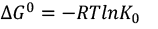(10)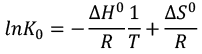(11)

T, the absolute temperature in Kelvin and R is universal gas constant (8.314J⁄(mol.K)). The thermodynamic distribution coefficient (K0) for the process of adsorption can be obtained as following :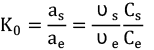(12)

Where, as and υs are, the activity and activity coefficient of the MB, respectively; and ae and υe are, respectively, the activity and the activity coefficient of the MB in the equilibrium solution. Csis the amount of MB adsorbed (mg) in every solvent liter in contact with the surface of the adsorbent. Ce is the amount of MB (mg) in every solvent liter in the equilibrium state. According to Biggar and Cheung , as the solute concentration in the solution approaches to zero, the coefficients of activity get the value equal to one, and therefore, Equation (12) can be defined as below: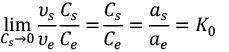(13)

K0 values can be estimated through plotting ln(Cs/Ce) vs. Cs and extrapolating Cs →0. In the present study, thermodynamic parameters were obtained from the variation of the lnK0 vs. 1/T. The MB adsorption’s thermodynamic parameters onto MFS are listed in Table 2a. The ∆G0 negative values show that the process of adsorption is spontaneous and highly favorable at low temperatures. The ∆S° negative value reflects the decreased randomness at the interface of solid/solution in the process of adsorption .

The ∆H0 value provides data about the effective forces on the process of adsorption. The energy is related to various physical forces including hydrophobic bond forces (5 kJ/mol), hydrogen bond forces (2–40 kJ/mol), van der Waals forces (4–10 kJ/mol), dipole bond forces (2–29 kJ/mol), coordination exchange (40 kJ/mol), and chemical forces (>60 kJ/mol) . ∆H0 was estimated equal to -38.299 kJ/mol, revealing that the physical forces including hydrogen bond forces and coordination exchange (in agreement with the results given in pH of point of zero charge section) affected the MB adsorption onto MFS. To confirm the fact that physical adsorption is the prominent mechanism, the sticking probability (S*) and activation energy (Ea) values were obtained from the data by employing an altered equation of Arrhenius type associated with the coverage of surface shown as following :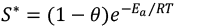(14)

Sticking probability and energy of activation (Table 2a) were obtained from the intercept and slope of plot Ln(1-θ) versus 1/T with the reasonable good fit (data not shown, R2>0.97); the surface coverage (θ) was calculated as below: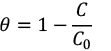(15)

C0 and C (mg/L) are, respectively, the initial and residual MB concentrations in the solution. The negative value of Ea indicates that the process of adsorption is basically exothermic. Relatively Ea low value proposes that adsorption is a diffusion controlled process. If 0<S*<1, then the adsorption process is physisorption. Eventually, according to the value of S* (0.7555), the MB adsorption process onto MFS is physisorption.

Fig. 11 and Table 2b represent the curves and fitting parameters of the calculated isotherms, respectively. The R2 values for the Langmuir, Temkin, Sips, and Redlich-Peterson models show desired fitting with the data. Compared with other models the Freundlich model represented the poorest fitting with the experimental data at all temperatures (0.93 < R< 0.96).

Fitting of isotherms can be expressed by the non-linear correlation coefficient (R2), however, it seems that an acceptable correlation coefficient is not enough for a model to be expressed as a consistent model by which describes the adsorption process. The adsorption nature must also be considered to indicate an appropriate model. As thermodynamic analysis indicated (adsorption thermodynamics section), the adsorption of MB onto MFS is exothermic. So even though the Langmuir model shows acceptable fitting with the data (R2 < 0.99), since the Langmuir maximum capacity of adsorption (Qmax) increases by increasing the temperature (Table 2b), it predicts the nature of the process inversely (endothermic). Since the Langmuir isotherm predicts adsorption for homogenous surfaces , thus the reason may be due to the heterogeneity of the adsorbent surface. On the other hand, the Sips model can achieve both a good fitting (R2 < 0.99) and a correct prediction of the adsorption process nature (exothermic) simultaneously.

The Beta parameter (β) value in the Redlich-Peterson model is basically between zero and one . When the value of β is equal to one, this equation become equalized to the Langmuir equation . But in this study, the beta parameters of the Redlich-Peterson model were bigger than one (Table 2b). One possible explanation may be that β > 1 can confirm the deviation of the Langmuir model from the characteristic of the adsorption process. Based on Table 2b, as the β value gets bigger than one, the Langmuir model shows more deviation from the nature of process.

The Freundlich constant n renders a favorability of adsorption measure. n > 1values show a desired process of adsorption . The value of n shows the same trend at all studied temperatures which shows the favorable nature characteristic of adsorption of MB by MFS. As it is clear from Table 2b, the value of n decreases by increasing the temperature; this confirms the adsorption’s exothermic nature which is concluded formerly by thermodynamics analysis. According to the best correlation coefficient and the above explanations, Sips isotherm is the best model for describing the process of adsorption of MB onto MFS.

The linear forms of the rate of pseudo-first and pseudo-second-order equations were analyzed, and their parameters are represented in Table 2c. The adsorption kinetics of MB onto MFS is suggested to be successfully simulated by the pseudo-second-order kinetic model (R2 < 0.99) in comparison with the pseudo-first-order kinetic model (R2 < 0.87). The constant of pseudo-second-order rate declined from 0.1324 to 0.0114 g/mg.min by raising the initial dye concentration from 50 to 200 mg/L.

The XRD patterns of adsorbent before and after the regeneration process is shown in Fig. 5c and d. The diffraction peaks at 2θ=26° and 32° were omitted after the regeneration process, which can be due to the extraction of minerals in FS by HCl. This conclusion can be approved by the EDX analysis (Table 1) where the atomic percent of all Na, Mg and Ca is almost equal to zero in RMFS.

Based on the EDX analysis, 75.19% of Fe was regenerated, which can approve the removal of MB from UMFS. The EDM analysis of Fe before and after regeneration of UMFS is shown in Fig. 12, where the white points represent Fe element. The increase of the atomic percent of Cl in RMFS (Table 1) is due to the regeneration of UMFS by HCl. The FESEM images before and after the regeneration process are shown in Fig. 3c and d.

It can be seen that that uniform layer of dye molecules in UMFS has been mostly cleared from the adsorbent surface, and the initial adsorbent’s mesoporous structure has appeared. The equilibrium study was conducted for the regenerated adsorbent at 303 K. Since the Sips isotherm was concluded to be the best model predicting the adsorption of MB onto MFS, it was chosen for equilibrium analysis. A comparison of the MB adsorption isotherm to MFS and RMFS with Sips model is shown in Fig. 11d. The Sips maximum adsorption capacity (Qmax) was obtained as 36.3 mg/g for the RMFS adsorbent. The efficiency of the regeneration process can be defined as:

(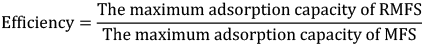16)

So it can be concluded that the efficiency of the regeneration process at 303 K is equal to 59.63 %.

The comparison between MFS’s maximum adsorption capacity (Qmax) and other low-cost adsorbents for MB adsorption is presented in Table 3. The results showed that both MFS and RMFS have an acceptable adsorption capacity compared to many other adsorbents, and can be suitable adsorbent candidates for water treatment.

CONCLUSION

The synthesis of a nano-magnetic FS was done by precipitation technique. The results showed a significant rise in the synthesized adsorbent’s special surface area. The FS and MFS’s specific surface areas were obtained, respectively, as 0.65 and 4.86 m2/g. The analysis of SEM showed that the adsorbent was homogenized and smoothed, and had less porosity after the adsorption of MB. The EDX analysis revealed that the FS composition mainly included C, O, P and Ca elements. The isotherm results and negative ∆H0 values suggest that the adsorption onto MFS is a physisorption process and exothermic in nature. The maximum adsorption capacity for the Sips model (best fitted isotherm) was 60.87 mg/g. The adsorption kinetics pursued the pseudo-second order kinetics model, and the regeneration process was achieved with the efficiency of 59.63%. Finally, an electrostatic interaction mechanism (among the negative groups on the MFS surface and positive groups of MB) was presented to explain the adsorption of MB onto MFS.

ACKNOWLEDGMENTS

The authors want to acknowledge from the University of Guilan and University of Tehran for the technical support.

CONFLICTS OF INTEREST

The authors declare that there are no conflicts of interest.